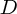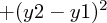## Distance

• Distance is a scalar quantity (compared with the vector quantity displacement) giving the magnitude of the measurement of how far apart two points are. In two-dimensional space, the distancebetween two pointsandis given by the formula